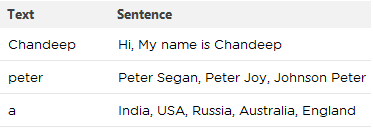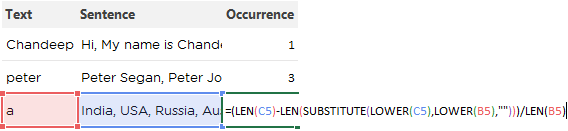This is a one odd problem that I was asked that how can we find occurrence of a word in a sentence. A simple formula can do it..

Let’s see how

### Consider this dataWe have to find how many times the text appears in the sentence?

### The formula`=(LEN(C5)-LEN(SUBSTITUTE(LOWER(C5),LOWER(B5),"")))/LEN(B5)`

Note that C5 is the sentence and B5 is the word / text

Decoding the formula

1. First the formula is turning everything into lower case for avoiding case sensitivity, using the LOWER function
2. The formula is taking off the word from the sentence. This is done by the SUBSTITUTE function
3. Then the formula is counting the Length of the sentence without the word, using the LEN function
4. The difference between the length of the full sentence and sentence without the word is calculated then divided by the length of the word. This is will give is how many times the word appeared in the sentence